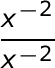# Common Core Math - Functions: High School Standards Final Exam

Free Practice Test Instructions:

Choose your answer to the question and click 'Continue' to see how you did. Then click 'Next Question' to answer the next question. When you have completed the free practice test, click 'View Results' to see your results. Good luck!

#### Question 2 2. What's the value of sec2 (θ) given that the equation below is true?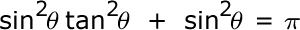#### Question 3 3. What's the value of 2sin(θ)cos(θ) given that the equation below is true?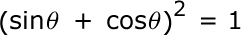#### Question 10 10. Simplify the following expression.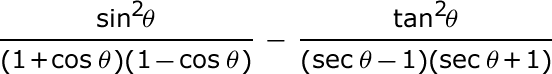#### Question 14 14. Convert the following measurement in radian to degrees.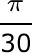#### Question 15 15. Simplify the following expression.